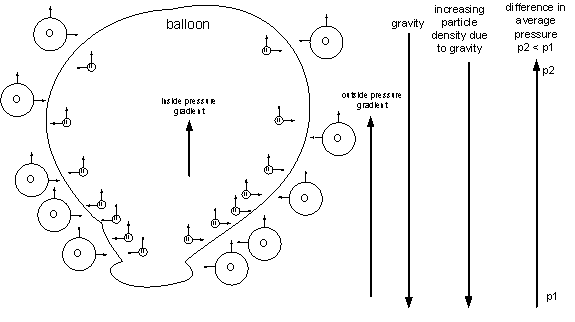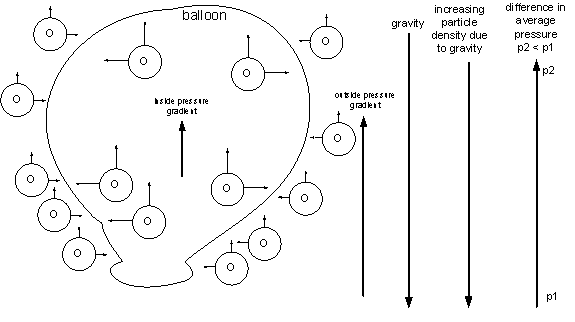I’ve always wondered why a helium balloon goes up.  The common answer is that helium is lighter than air.  However, this doesn’t explain what invisible force is pushing the balloon higher.  To say that helium is lighter than air is true.  The individual helium atoms are smaller than the constituent atoms and molecules of air.

Air is made of atoms and molecules of different sizes and weights but it still remains a fairly homogeneous gaseous soup.  Why, for example, wouldn’t the nitrogen atoms (atomic number 7, 78% of the mix), rise into space, being lighter than the heavier, though not by much, oxygen atoms (atomic number 8, 20% of the mix)?  Helium’s atomic number is 2.  If this were the case, wouldn’t air stratify into layers, the heaviest below and lightest above?  A similar problem is why air bubbles rise in water.  The stock answer is, there is a force of buoyancy proportional to the volume of water displaced.  But this does not explain why a rock, while feeling lighter underwater, fails to rise, or why huge ships weighing thousand of tons, float like feathers.

Another possibility is that the balloon operates under the same principle of the golf ball in a container of beans.  When shaken, the golf ball rises to the top.  An analogy used is that the golf ball is you and the beans are your problems.  Why does the ball rise?  Because you are bigger than your problems.  But this says nothing of the lightness of the ball relative to the beans.  The ball is obviously heavier than an individual bean.

The answer to the problem can be found if we reduce it to a very simple level as illustrated by the following figure:The balloon contains helium atoms, which are smaller and have less mass than the larger oxygen atoms outside the balloon.  Gravity pushes the atoms downward and the density of atoms increases at lower levels.  As the density increases, the total upward force due to the atoms hitting the balloon increases, which means that the total upward force decreases as you go higher.  The helium atoms apply a smaller upward force due to their smaller mass so the pressure gradient inside the balloon in less than the pressure gradient outside the balloon.  A greater force at the lower level pushes against the lesser force at the higher level, resulting in a net force upward.

In the case where the balloon is filled with hotter oxygen atoms, the more energetic atoms inside the balloon tend less to gather at the bottom of the balloon then the cooler outside atoms resulting in less particle density inside the balloon.  This means, again, that the upward pressure gradient inside the balloon is less than that outside the balloon and the balloon rises.Is the shape of the balloon is also important?  If the balloon were long and thin, would there would be no pressure pushing the balloon upward?  Would all the pressure be concentrated in the horizontal, rather than the vertical direction?It turns out that even on a vertical surface there is a net upward force on the surface applied by the atom at the point of contact.  This is one of the odd things about vectors (the thicker arrows in the diagram) in that the upward force appears to be pushing up against nothing, i.e. vertically alone the surface, not against it.  But there still is a upward force applied to the surface because the angle at which the oxygen atom hits it creates an upward force component of the vector.  Where is this force applied?  There’s only one place: the point of contact.

Question: So if there is a pressure gradient, why doesn’t the balloon rise when there is air inside it that’s the same temp as the outside?  There would be the same pressure gradient inside the balloon which should double the upward force, true?

Actually, a balloon with air in it is “lighter” that a balloon without air.  Try dropping an empty balloon and a balloon with air in it and the balloon filled with air will drop at a slower rate than the empty balloon.  This is the result of the gradient pressures inside and outside the balloon which create buoyancy.  You see the same effect underwater, where the water molecules push against an object just as a balloon in the atmosphere.  Think of the water as being the same thing as as the atmosphere, but with larger particles, i.e. the water molecules providing the force.  Objects underwater are lighter than in air because of this effect.

When the balloon is filled with air, the air molecules apply the same force both up and down, but mostly up, on the balloon, making the balloon lighter, but providing a total pressure gradient (the sum of both inside and outside gradients) that is insufficient to make the balloon rise.  Only when the inside down pressure gradient is lower, does the total down pressure gradient decrease to the point that the up pressure gradient pushes the balloon higher.  This happens when the total down momentum of the inside gas (mass x velocity) is lower.